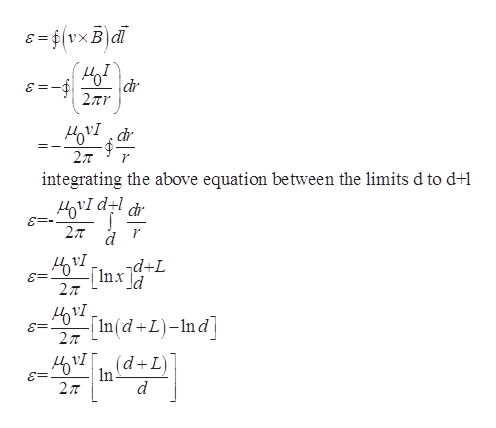Question
32 views

29.57     The long, straight wire shown in Fig. P29.57a carries constant current I.  A metal bar with length L is moving at constant velocity v, as shown in the figure.  Point a is a distance d from the wire.

(a) Calculate the emf induced in the bar.

(b) Which point, a or b, is at higher potential?

(c) If the bar is replaced by a rectangular wire loop of resistance R (Fig. P29.57b), what is the magnitude of the current induced in the loop?

Hint: (b) and (c) are conceptual questions.

check_circle

Step 1

a)

As shown from the diagram, divide the total length of the rod into small number of elements dx which is at a distance x from current carrying wire.

Magnitude of magnetic field due to current carrying ire at a distance x from the element is

Step 2

The induced emf in a rod moves normal to magnetic field ishelp_outlineImage TranscriptioncloseE=6(VXB|d dr 2Tr ovIar 2л integrating the above equation be tween the limits d to d+ 2T r d d+L -[1nx \d 2л In(d +L)-Ind 2л (d In -L) fullscreen
Step 3

b)

The magnitude of force acting on moving...

### Want to see the full answer?

See Solution

#### Want to see this answer and more?

Solutions are written by subject experts who are available 24/7. Questions are typically answered within 1 hour.*

See Solution
*Response times may vary by subject and question.
Tagged in

### Physics